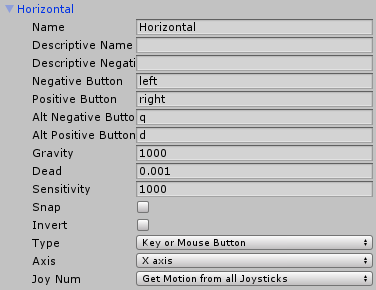# Input axis : both positive and negative pressed simultaneously

Hello !

If I setup an axis with two button (positive and negative) and press both, I have an unexpected behaviour :
GetAxis() return the value of the first button pressed, the second button seems to be ignored.

I thought that pressed the two buttons at the same time will produce zero.
Is there a way to setup the axis to work this way ? Or I have to manage this in my script ?

UPDATE

As requested, a screenshot and a piece of code :``````float xAxis = Input.GetAxis("Horizontal");
``````

Not sure if you’ve found a solution yet but if anyone else is stuck try using Input.GetAxisRaw(). Keep in mind that GetAxisRaw() does not smooth input values, so it will return a value of either 1, -1 or 0, whereas GetAxis() will return values in-between 1 and -1 (or 0 if no input is detected).

This solution worked perfectly for my very basic FPS movement script:

``````using UnityEngine;

public class FPS_Move : MonoBehaviour
{
public float _force, _maxSpeed;

[SerializeField]
private float _currentSpeed, z, x;

private Vector3 _direction;
private Rigidbody _rBody;

void Start()
{
_direction = Vector3.zero;
_rBody = GetComponent<Rigidbody>();
}

void FixedUpdate()
{
//For Debugging
_currentSpeed = _rBody.velocity.magnitude;
if (_currentSpeed < 0)
{
_currentSpeed = 0;
}

x = Input.GetAxisRaw("Horizontal");
z = Input.GetAxisRaw("Vertical");

if (x != 0 || z != 0)
{
_direction = transform.right * x + transform.forward * z;
_direction.Normalize();